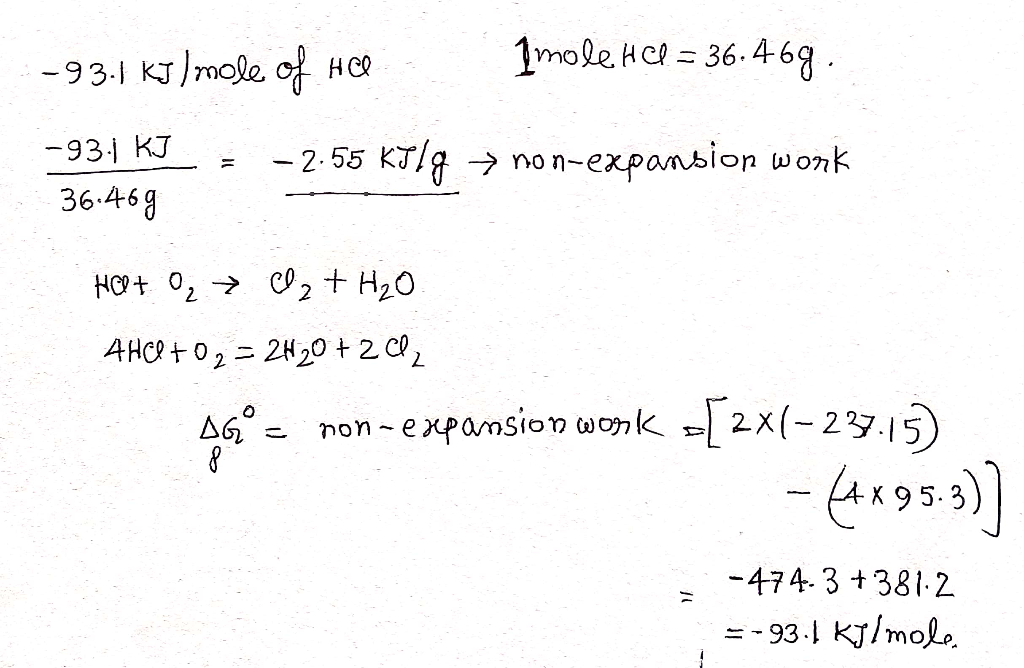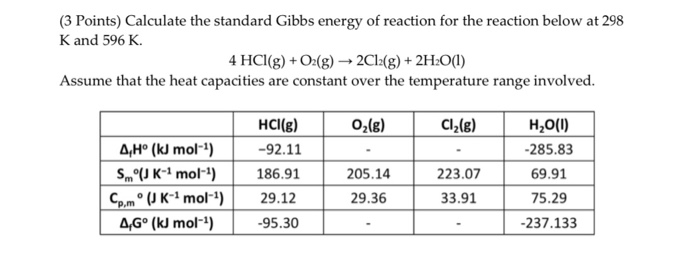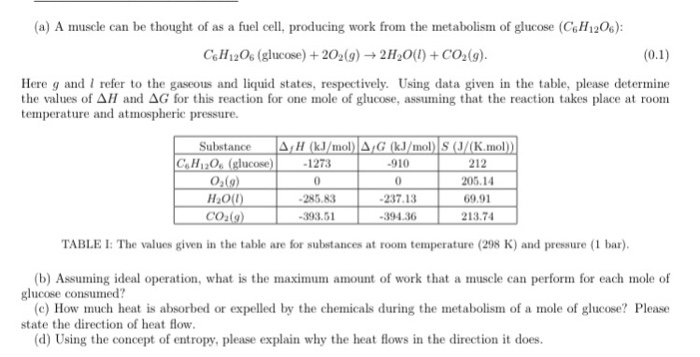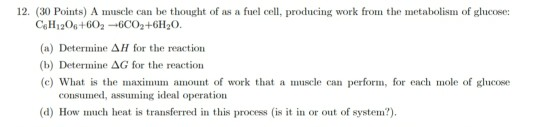# Calculate the maximum nonexpansion work that can be gained from the oxidation of HCl (g) on...

Calculate the maximum nonexpansion work that can be gained from the oxidation of HCl (g) on a per gram basis under standard conditions. Please answer your question (unit kJ/g).

 HCl (g) O2 (g) Cl2 (g) H2O (l) ∆fHo(kJmol-1) -92.31 0 0 -285.83 So (JK-1mol-1) 186.91 205.14 223.07 69.91 ∆fGo (kJmol-1) -95.3 0 0 -237.15

I got until the point where you get kJ/m my answer was -93.1. But, from there I don't know what is the next step to convert to kJ/g. I tried and I got the answer -23.04 but that was wrong.

Please show me step by step, and result.

Thank you.#### Earn Coin

Coins can be redeemed for fabulous gifts.

Similar Homework Help Questions
• ### (3 Points) Calculate the standard Gibbs energy of reaction for the reaction below at 298 K...(3 Points) Calculate the standard Gibbs energy of reaction for the reaction below at 298 K and 596 K. 4 HCl(g) + O2(g) 2Cl2(g) + 2H2O(l) Assume that the heat capacities are constant over the temperature range involved. 02(g) Cl2(g) HCl(g) 4,Hº (kJ mol-4) -92.11 S JK- mol-) 186.91 Comº (K-mol-4) 29.12 AG° (k mol-1) | -95.30 223.07 205.14 9.36 H2O(1) -285.83 69.91 75.29 -237.133 2 33.91

• ### (a) A muscle can be thought of as a fuel cell, producing work from the metabolism...(a) A muscle can be thought of as a fuel cell, producing work from the metabolism of glucose (C6H1206): C6H1206 (glucose) +202(9) + 2H2O(l) + CO2(g). (0.1) Here g and I refer to the gaseous and liquid states, respectively. Using data given in the table, please determine the values of AH and AG for this reaction for one mole of glucose, assuming that the reaction takes place at room temperature and atmospheric pressure. Substance CH 20. (glucose) 0 (9) H200...

• ### 12. (30 Points) A muscle can be thought of as a fuel cell, producing work from...12. (30 Points) A muscle can be thought of as a fuel cell, producing work from the metabolism of glucose C.H1206+603-6002+6H20. (a) Determine AH for the reaction (1) Determine AG for the reaction (c) What is the maximum amount of work that a muscle can perform, for each mole of glucose consumed, assuming ideal operation (d) How much heat is transferred in this process is it in or out of system?). IRUN II 30), LKR ), and Anderson (1996). Please...